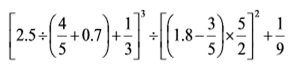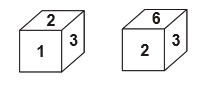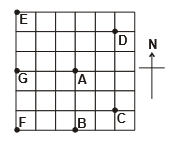# CREST Mathematics Olympiad Class 5 Sample Papers

## Syllabus:

Section 1: Numerals, Number Names and Number Sense (7 and 8 digit numbers), Computation Operations, Fractions and Decimals, Measurement of Length, Weight, Capacity, Volume, Time, Temperature and Money, Conversions, Geometrical Shapes and Solids, Angles, Perimeter of Various Shapes & Area of Rectangle and Square, Symmetry, Data Handling.

Achievers Section: Higher Order Thinking Questions - Syllabus as per Section 1

 Q.1 An electrician is paid Rs. 14.30 per hour for a 40-hour week. She is paid time and a half for overtime (1.5 times the hourly wage) for every hour more than 40 hours worked in the same week. If she works 48 hours in one week, what will she earn for that week?
 Q.2 Solve.Q.3 Fred has a rectangular living room that is 3.5 yards wide and 6.8 yards long. He has a hallway that is 1.8 yards wide and 3.5 yards long. He wants to carpet each area using carpeting that costs Rs.75 per square yard. What will the carpeting cost him?
 Q.4 If P means subtraction, M means addition, S means multiplication and Q means division then, 34 M 1 S 2 P 24 Q 8 = ?
 Q.5 7 million - 500 thousand is equal to _______.
 Q.6 A representative sample of Secondary Schools in Uttar Pradesh took part in the 2016 Census School. The mean number of schools per province for 8 of the 9 provinces was 78. What is the total number of schools in the 8 provinces that took part in Census School?
 Q.7 A package of 10 small envelopes costs Rs. 5, and a package of 10 large ones costs 2/5 more. Find the total cost of buying 50 envelopes of each kind.
 Q.8 Which digit will appear on the face opposite to the face with number 1?Q.9 If "ICECREAM" is coded as "CICEERMA' then "CHOCOLATES" will be coded as  _______.
 Q.10 Which point is south­east of A?Sample PDF of CREST Mathematics Olympiad for Class 5: